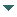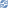# gvSIG

##### Personal tools
You are here: Home Geometry register
gvSIG DesktopgvSIG Desktop

Cached time 11/21/13 11:24:39# Geometry register

Up one level
 ◄ Previous Geometry type Next: Geometry creation ►

This document explain how to register the geometry types

All the geometries that you want to use in the application must have a type (GeometryType) previously registered. If we try to create a geometry with a non-registered type in the GeometryManager, we will get an exception of the kind CreateGeometryException.

To register a new geometry type, first of all we must obtain an instance of the GeometryManager and, later, we will register the implementation of the geometry type. To do this, we must know the geometry type and subtype. The diferent kinds of types are defined on TYPES, and the subtypes in SUBTYPES.

In the next example we show how to register a 2-dimensional point (is assumed that exists a Point2D class which inherits from Geometry).

```geometryManager.registerGeometryType(Point2D.class, "Point2D", TYPES.POINT, SUBTYPES.GEOM2D);
```

The method registerGeometryType has 4 parameters. First of them is the class which contains the geometry implementation; the second one is the name to register and, the third and fourth, the geometry type and subtype.

In this case, is registering the default geometry for the type 2-dimensional point. When we invoke this method, the manager will associate to the specified type and subtype the implementation of the geometry that we have passed in first parameter, so that, when we create a geometry with that types, the manager will return an instance of the Point2D class.

The next example shows how to register a 3-dimensional point. The example assums that exists a Point3D class which inherits from Geometry and implements a 3-dimensional point:

```geometryManager.registerGeometryType(Point3D.class, "Point3D", TYPES.POINT, SUBTYPES.GEOM3D);
```

In this other example, we register a geometry of the circle type. The example assums that exists a Circle2D class which inherits from Geometry and implements a 2-dimensional circle:

```geometryManager.registerGeometryType (Circle2D.class, "Circle2D", TYPES.CIRCLE, SUBTYPES.GEOM2D);
```
 ◄ Previous Geometry type Next: Geometry creation ►